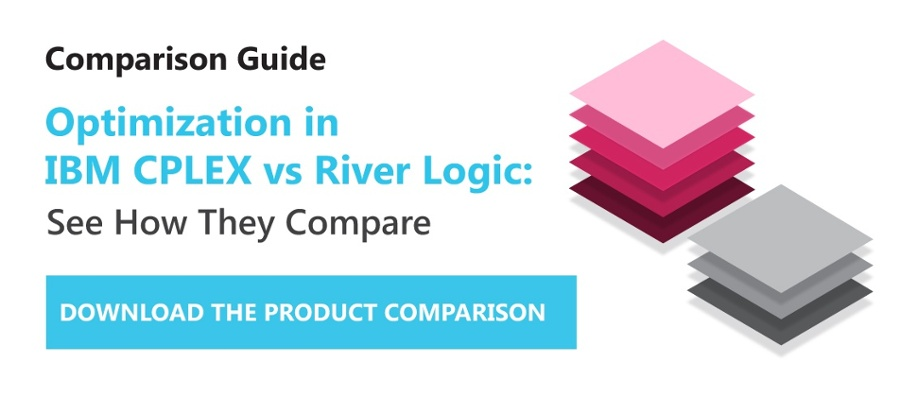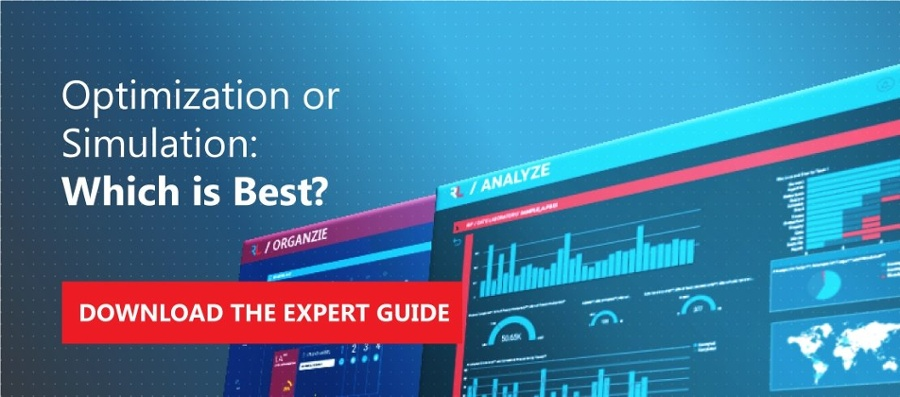# What Is the Difference Between Optimization Modeling and Simulation?

Engineers have used models for decades to help them understand processes and determine optimal solutions. Sometimes models are created so designers discover answers to specific design problems, and in other circumstances, they’re used to simulate how the model performs in different situations. Physical modeling processes persisted until late in the 20th century when the development of modeling software allowed engineers to more readily explore model performance using virtual modeling.

Although outwardly similar, simulation and modeling processes are distinctly different. In simulation, an analyst runs multiple scenarios to predict how a system or process performs under different conditions, and it's the basis for predictive analytics.

Modeling, also known as optimization modeling, differs in that it can determine a specific, optimal or best outcome of a specific scenario. This is known as prescribing an outcome, hence the name prescriptive analytics.

Let's learn more about the difference between modeling and simulation.

## Definition of Modeling

A model is a representation of a physical object or process. The model may be physical or mathematical and closely replicates the object or process. It may be used to determine answers to questions such as which is the best business decision to make given a particular set of circumstances.

A key aspect of optimization modeling (mathematical) is the use of mathematical equations and techniques to create models that perform in much the same way as the process being modeled. This applies as much to a business model as it does to a CAD model that incorporates formulae representing the physical strength and rigidity of structural components.

### Modeling Examples

As mentioned, modeling can be performed with both physical and mathematical models. For example, Formula 1 racing car teams create exact scale models of their cars to determine the best arrangement of various aero components to minimize drag at high speed or to increase downforce.

In the business environment, supply chain managers may use optimization modeling for supply chain network design to determine the best mix of suppliers, locations, logistics and production for optimal supply. Such an approach models the supply chain, then populates the model with organizational and other data, using solver software to determine, in specific financial terms, financial returns to facilitate correct decisions.## What Is Simulation?

The dictionary definition of simulation refers to two specific processes: that of representing how a system functions by creating a representation of the system or the examination of a problem by simulating a real-life situation.

In other words, it relates to a study of the behavior and performance of a real-world system through testing a theoretical, mathematical or physical representation of the system. Through the application of different conditions, it's possible to discover and understand how a real-life system performs. Simulation is used to gain insight into how the system performs under different scenarios.

### Simulation examples

In the Second World War, Britain created an exact scale model of the Mohne Dam in the Ruhr Valley in Germany. They used it to study how to bounce massive spherical bombs across the surface of the water so they fell to the bottom of the dam wall before exploding. They also simulated dropping full-sized practice bombs to establish the correct speed, altitude and position needed to drop the bombs correctly.

Weather forecasters use simulation models to predict future weather. These predictions allow organizers of outdoor events to prepare for likely weather as well as letting forecasters give warning of possible abnormal weather. While weather forecasting is often remarkably accurate, based on multiple simulations, it's nevertheless incapable of providing specific answers except possibly very close to the time.

## Difference Between Optimization Modeling and Simulation

It's important to understand that each approach is valid, offering differing insights and information.

The key difference between modeling and simulation is that optimization modeling provides a definite recommendation for action in a specific situation, while simulation allows users to determine how a system responds to different inputs so as to better understand how it operates.

In optimization modeling, mathematical techniques are used to represent a real-world situation. This approach takes into account key variables, constraints and trade-offs so as to determine an optimal solution that's feasible and achieves certain objectives.

Simulation, on the other hand, evaluates multiple different scenarios in an attempt to understand how a system would work. A good example is the Monte Carlo simulation that uses randomly-generated inputs based on probability to determine the outcome of various scenarios. Limitations of this approach are that outcomes may not be feasible or even workable. Additionally, it isn't possible to accurately determine the single best optimal solution, as answers are dependent upon the randomly generated variables, although in some instances answers can come close.## Using Optimization Modeling and Simulation Together

The differences between optimization modeling and simulation can come in handy at times. While optimization modeling will recommend specific strategies, simulation can be used for optimizing scenario planning and help answer what-if questions.

Another way to exploit these differences is to use simulation to broadly understand how a system works before using optimization modeling to determine specific answers. For example, an organization may use simulation modeling to determine whether there's a need for an additional distribution center and optimization modeling to determine its optimal location and size.

## Gaining Greater Certainty by Exploiting the Difference Between Modeling and Simulation.

Simulation analysis is useful for predicting future performance. Sales executives use predictive analytics to simulate future market trends and changes, while stockbrokers use it to anticipate changes in stock and bond indices. Optimization modeling takes this capability a step further with its ability to provide specific, data-driven answers to specific business problems.

True optimization modeling, the basis for prescriptive analytics software such as River Logic's Enterprise Optimizer, would always use data derived from descriptive and prescriptive analytics to determine optimal solutions to specific business problems and, as a tool, to analyze different scenarios.You May Also Enjoy Reading